# Survival Analysis with Plotly in Python/v3

An introduction to survival analysis with Plotly graphs using R, Python, and IPython notebooks

See our Version 4 Migration Guide for information about how to upgrade.

In this notebook, we introduce survival analysis and we show application examples using both R and Python. We will compare the two programming languages, and leverage Plotly's Python and R APIs to convert static graphics into interactive plotly objects.

Plotly is a platform for making interactive graphs with R, Python, MATLAB, and Excel. You can make graphs and analyze data on Plotly’s free public cloud. For collaboration and sensitive data, you can run Plotly on your own servers.

For a more in-depth theoretical background in survival analysis, please refer to these sources:

Need help converting Plotly graphs from R or Python?

For this code to run on your machine, you will need several R and Python packages installed.

• Running sudo pip install <package_name> from your terminal will install a Python package.

• Running install.packages("<package_name>") in your R console will install an R package.

You will also need to create an account with Plotly to receive your API key.

In :
# You can also install packages from within IPython!

# Install Python Packages
!pip install lifelines
!pip install rpy2
!pip install plotly
!pip install pandas

# Load extension that let us use magic function %R

# Install R packages
%R install.packages("devtools")
%R devtools::install_github("plotly/plotly.R")
%R install.packages("OIsurv")


## Introduction¶

Survival analysis is a set of statistical methods for analyzing the occurrence of events over time. It is also used to determine the relationship of co-variates to the time-to-events, and accurately compare time-to-event between two or more groups. For example:

• Time to death in biological systems.
• Failure time in mechanical systems.
• How long can we expect a user to be on a website / service?
• Time to recovery for lung cancer treatment.

The statistical term 'survival analysis' is analogous to 'reliability theory' in engineering, 'duration analysis' in economics, and 'event history analysis' in sociology.

The two key functions in survival analysis are the survival function and the hazard function.

The survival function, conventionally denoted by $S$, is the probability that the event (say, death) has not occurred yet:

$$S(t) = Pr(T > t),$$

where $T$ denotes the time of death and $Pr$ the probability. Since $S$ is a probability, $0\leq S(t)\leq1$. Survival times are non-negative ($T \geq 0$) and, generally, $S(0) = 1$.

The hazard function $h(t)$ is the event (death) rate at time $t$, conditional on survival until $t$ (i.e., $T \geq t$):

\begin{align*} h(t) &= \lim_{\Delta t \to 0} Pr(t \leq T \leq t + \Delta t \, | \, T \geq t) \\ &= \lim_{\Delta t \to 0} \frac{Pr(t \leq T \leq t + \Delta t)}{S(t)} = \frac{p(t)}{S(t)}, \end{align*}

where $p$ denotes the probability density function.

In practice, we do not get to observe the actual survival function of a population; we must use the observed data to estimate it. A popular estimate for the survival function $S(t)$ is the Kaplan–Meier estimate:

\begin{align*} \hat{S}(t) &= \prod_{t_i \leq t} \frac{n_i − d_i}{n_i}\,, \end{align*}

where $d_i$ is the number of events (deaths) observed at time $t_i$ and $n_i$ is the number of subjects at risk observed at time $t_i$.

## Censoring¶

Censoring is a type of missing data problem common in survival analysis. Other popular comparison methods, such as linear regression and t-tests do not accommodate for censoring. This makes survival analysis attractive for data from randomized clinical studies.

In an ideal scenario, both the birth and death rates of a patient is known, which means the lifetime is known.

Right censoring occurs when the 'death' is unknown, but it is after some known date. e.g. The 'death' occurs after the end of the study, or there was no follow-up with the patient.

Left censoring occurs when the lifetime is known to be less than a certain duration. e.g. Unknown time of initial infection exposure when first meeting with a patient.

For following analysis, we will use the lifelines library for python, and the survival package for R. We can use rpy2 to execute R code in the same document as the python code.

In :
# OIserve contains the survival package and sample datasets
%R library(OIsurv)
%R library(devtools)
%R library(plotly)
%R library(IRdisplay)

# Authenticate to plotly's api using your account
%R py <- plotly("rmdk", "0sn825k4r8")

import numpy as np
import pandas as pd
import lifelines as ll

# Plotting helpers
from IPython.display import HTML
%matplotlib inline
import matplotlib.pyplot as plt
import plotly.plotly as py
import plotly.tools as tls
from plotly.graph_objs import *

from pylab import rcParams
rcParams['figure.figsize']=10, 5

Loading required package: survival

Loading required package: RCurl


We will be using the tongue dataset from the KMsurv package in R, then convert the data into a pandas dataframe under the same name.

This data frame contains the following columns:

• type: Tumor DNA profile (1=Aneuploid Tumor, 2=Diploid Tumor)
• time: Time to death or on-study time, weeks
• delta Death indicator (0=alive, 1=dead)
In :
# Load in data
%R data(tongue)
# Pull data into python kernel
%Rpull tongue
# Convert into pandas dataframe
from rpy2.robjects import pandas2ri

tongue = pandas2ri.ri2py_dataframe(tongue)


We can now refer to tongue using both R and python.

In :
%%R
summary(tongue)

      type           time            delta
Min.   :1.00   Min.   :  1.00   Min.   :0.0000
1st Qu.:1.00   1st Qu.: 23.75   1st Qu.:0.0000
Median :1.00   Median : 69.50   Median :1.0000
Mean   :1.35   Mean   : 73.83   Mean   :0.6625
3rd Qu.:2.00   3rd Qu.:101.75   3rd Qu.:1.0000
Max.   :2.00   Max.   :400.00   Max.   :1.0000

In :
tongue.describe()

Out:
type time delta
count 80.000000 80.000000 80.00000
mean 1.350000 73.825000 0.66250
std 0.479979 67.263091 0.47584
min 1.000000 1.000000 0.00000
25% 1.000000 23.750000 0.00000
50% 1.000000 69.500000 1.00000
75% 2.000000 101.750000 1.00000
max 2.000000 400.000000 1.00000

We can even operate on R and Python within the same code cell.

In :
%R print(mean(tongue$time)) print tongue['time'].mean()   73.825  73.825  In R we need to create a Surv object with the Surv() function. Most functions in the survival package apply methods to this object. For right-censored data, we need to pass two arguments to Surv(): 1. a vector of times 2. a vector indicating which times are observed and censored In : %%R attach(tongue) tongue.surv <- Surv(time[type==1], delta[type==1]) tongue.surv    1 3 3 4 10 13 13 16 16 24 26 27 28 30 30  32 41 51 65 67 70 72 73 77 91 93 96 100 104 157  167 61+ 74+ 79+ 80+ 81+ 87+ 87+ 88+ 89+ 93+ 97+ 101+ 104+ 108+  109+ 120+ 131+ 150+ 231+ 240+ 400+  • The plus-signs identify observations that are right-censored. # Estimating survival with Kaplan-Meier¶ ### Using R¶ The simplest fit estimates a survival object against an intercept. However, the survfit() function has several optional arguments. For example, we can change the confidence interval using conf.int and conf.type. See help(survfit.formula) for the comprehensive documentation. In : %%R surv.fit <- survfit(tongue.surv~1) surv.fit  Call: survfit(formula = tongue.surv ~ 1) records n.max n.start events median 0.95LCL 0.95UCL 52 52 52 31 93 67 NA  It is often helpful to call the summary() and plot() functions on this object. In : %%R summary(surv.fit)  Call: survfit(formula = tongue.surv ~ 1) time n.risk n.event survival std.err lower 95% CI upper 95% CI 1 52 1 0.981 0.0190 0.944 1.000 3 51 2 0.942 0.0323 0.881 1.000 4 49 1 0.923 0.0370 0.853 0.998 10 48 1 0.904 0.0409 0.827 0.988 13 47 2 0.865 0.0473 0.777 0.963 16 45 2 0.827 0.0525 0.730 0.936 24 43 1 0.808 0.0547 0.707 0.922 26 42 1 0.788 0.0566 0.685 0.908 27 41 1 0.769 0.0584 0.663 0.893 28 40 1 0.750 0.0600 0.641 0.877 30 39 2 0.712 0.0628 0.598 0.846 32 37 1 0.692 0.0640 0.578 0.830 41 36 1 0.673 0.0651 0.557 0.813 51 35 1 0.654 0.0660 0.537 0.797 65 33 1 0.634 0.0669 0.516 0.780 67 32 1 0.614 0.0677 0.495 0.762 70 31 1 0.594 0.0683 0.475 0.745 72 30 1 0.575 0.0689 0.454 0.727 73 29 1 0.555 0.0693 0.434 0.709 77 27 1 0.534 0.0697 0.414 0.690 91 19 1 0.506 0.0715 0.384 0.667 93 18 1 0.478 0.0728 0.355 0.644 96 16 1 0.448 0.0741 0.324 0.620 100 14 1 0.416 0.0754 0.292 0.594 104 12 1 0.381 0.0767 0.257 0.566 157 5 1 0.305 0.0918 0.169 0.550 167 4 1 0.229 0.0954 0.101 0.518  In : %%R -h 400 plot(surv.fit, main='Kaplan-Meier estimate with 95% confidence bounds', xlab='time', ylab='survival function')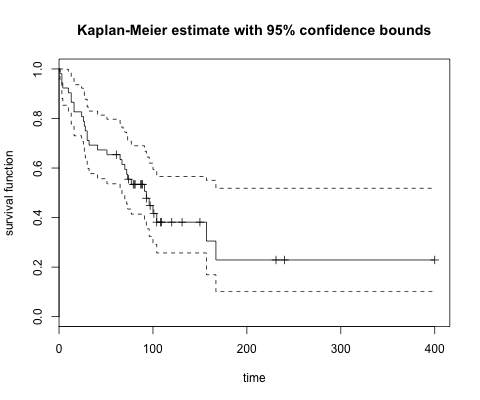Let's convert this plot into an interactive plotly object using plotly and ggplot2. First, we will use a helper ggplot function written by Edwin Thoen to plot pretty survival distributions in R. In : %%R ggsurv <- function(s, CI = 'def', plot.cens = T, surv.col = 'gg.def', cens.col = 'red', lty.est = 1, lty.ci = 2, cens.shape = 3, back.white = F, xlab = 'Time', ylab = 'Survival', main = ''){ library(ggplot2) strata <- ifelse(is.null(s$strata) ==T, 1, length(s$strata)) stopifnot(length(surv.col) == 1 | length(surv.col) == strata) stopifnot(length(lty.est) == 1 | length(lty.est) == strata) ggsurv.s <- function(s, CI = 'def', plot.cens = T, surv.col = 'gg.def', cens.col = 'red', lty.est = 1, lty.ci = 2, cens.shape = 3, back.white = F, xlab = 'Time', ylab = 'Survival', main = ''){ dat <- data.frame(time = c(0, s$time),
surv = c(1, s$surv), up = c(1, s$upper),
low = c(1, s$lower), cens = c(0, s$n.censor))
dat.cens <- subset(dat, cens != 0)

col <- ifelse(surv.col == 'gg.def', 'black', surv.col)

pl <- ggplot(dat, aes(x = time, y = surv)) +
xlab(xlab) + ylab(ylab) + ggtitle(main) +
geom_step(col = col, lty = lty.est)

pl <- if(CI == T | CI == 'def') {
pl + geom_step(aes(y = up), color = col, lty = lty.ci) +
geom_step(aes(y = low), color = col, lty = lty.ci)
} else (pl)

pl <- if(plot.cens == T & length(dat.cens) > 0){
pl + geom_point(data = dat.cens, aes(y = surv), shape = cens.shape,
col = cens.col)
} else if (plot.cens == T & length(dat.cens) == 0){
stop ('There are no censored observations')
} else(pl)

pl <- if(back.white == T) {pl + theme_bw()
} else (pl)
pl
}

ggsurv.m <- function(s, CI = 'def', plot.cens = T, surv.col = 'gg.def',
cens.col = 'red', lty.est = 1, lty.ci = 2,
cens.shape = 3, back.white = F, xlab = 'Time',
ylab = 'Survival', main = '') {
n <- s$strata groups <- factor(unlist(strsplit(names (s$strata), '='))[seq(2, 2*strata, by = 2)])
gr.name <-  unlist(strsplit(names(s$strata), '=')) gr.df <- vector('list', strata) ind <- vector('list', strata) n.ind <- c(0,n); n.ind <- cumsum(n.ind) for(i in 1:strata) ind[[i]] <- (n.ind[i]+1):n.ind[i+1] for(i in 1:strata){ gr.df[[i]] <- data.frame( time = c(0, s$time[ ind[[i]] ]),
surv = c(1, s$surv[ ind[[i]] ]), up = c(1, s$upper[ ind[[i]] ]),
low = c(1, s$lower[ ind[[i]] ]), cens = c(0, s$n.censor[ ind[[i]] ]),
group = rep(groups[i], n[i] + 1))
}

dat <- do.call(rbind, gr.df)
dat.cens <- subset(dat, cens != 0)

pl <- ggplot(dat, aes(x = time, y = surv, group = group)) +
xlab(xlab) + ylab(ylab) + ggtitle(main) +
geom_step(aes(col = group, lty = group))

col <- if(length(surv.col == 1)){
scale_colour_manual(name = gr.name, values = rep(surv.col, strata))
} else{
scale_colour_manual(name = gr.name, values = surv.col)
}

pl <- if(surv.col != 'gg.def'){
pl + col
} else {pl + scale_colour_discrete(name = gr.name)}

line <- if(length(lty.est) == 1){
scale_linetype_manual(name = gr.name, values = rep(lty.est, strata))
} else {scale_linetype_manual(name = gr.name, values = lty.est)}

pl <- pl + line

pl <- if(CI == T) {
if(length(surv.col) > 1 && length(lty.est) > 1){
stop('Either surv.col or lty.est should be of length 1 in order
to plot 95% CI with multiple strata')
}else if((length(surv.col) > 1 | surv.col == 'gg.def')){
pl + geom_step(aes(y = up, color = group), lty = lty.ci) +
geom_step(aes(y = low, color = group), lty = lty.ci)
} else{pl +  geom_step(aes(y = up, lty = group), col = surv.col) +
geom_step(aes(y = low,lty = group), col = surv.col)}
} else {pl}

pl <- if(plot.cens == T & length(dat.cens) > 0){
pl + geom_point(data = dat.cens, aes(y = surv), shape = cens.shape,
col = cens.col)
} else if (plot.cens == T & length(dat.cens) == 0){
stop ('There are no censored observations')
} else(pl)

pl <- if(back.white == T) {pl + theme_bw()
} else (pl)
pl
}
pl <- if(strata == 1) {ggsurv.s(s, CI , plot.cens, surv.col ,
cens.col, lty.est, lty.ci,
cens.shape, back.white, xlab,
ylab, main)
} else {ggsurv.m(s, CI, plot.cens, surv.col ,
cens.col, lty.est, lty.ci,
cens.shape, back.white, xlab,
ylab, main)}
pl
}


Voila!

In :
%%R -h 400
p <- ggsurv(surv.fit) + theme_bw()
p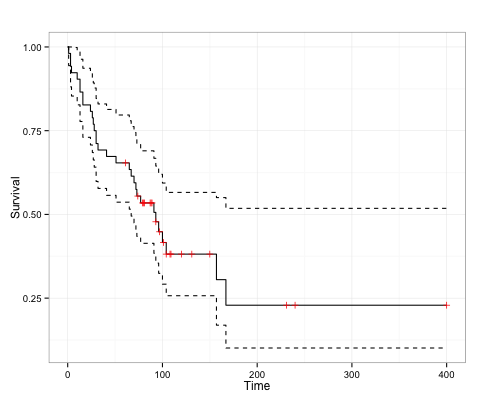We have to use a workaround to render an interactive plotly object by using an iframe in the ipython kernel. This is a bit easier if you are working in an R kernel.

In :
%%R
# Create the iframe HTML
plot.ly <- function(url) {
# Set width and height from options or default square
w <- "750"
h <- "600"
html <- paste("<center><iframe height=\"", h, "\" id=\"igraph\" scrolling=\"no\" seamless=\"seamless\"\n\t\t\t\tsrc=\"",
url, "\" width=\"", w, "\" frameBorder=\"0\"></iframe></center>", sep="")
return(html)
}

In :
%R p <- plot.ly("https://plotly.com/~rmdk/111/survival-vs-time/")
# pass object to python kernel
%R -o p

# Render HTML
HTML(p)

Out:

The y axis represents the probability a patient is still alive at time $t$ weeks. We see a steep drop off within the first 100 weeks, and then observe the curve flattening. The dotted lines represent the 95% confidence intervals.

### Using Python¶

We will now replicate the above steps using python. Above, we have already specified a variable tongues that holds the data in a pandas dataframe.

In :
from lifelines.estimation import KaplanMeierFitter
kmf = KaplanMeierFitter()


The method takes the same parameters as it's R counterpart, a time vector and a vector indicating which observations are observed or censored. The model fitting sequence is similar to the scikit-learn api.

In :
f = tongue.type==1
T = tongue[f]['time']
C = tongue[f]['delta']

kmf.fit(T, event_observed=C)

Out:
<lifelines.KaplanMeierFitter: fitted with 52 observations, 21 censored>

To get a plot with the confidence intervals, we simply can call plot() on our kmf object.

In :
kmf.plot(title='Tumor DNA Profile 1')

Out:
<matplotlib.axes._subplots.AxesSubplot at 0x10cff2e10>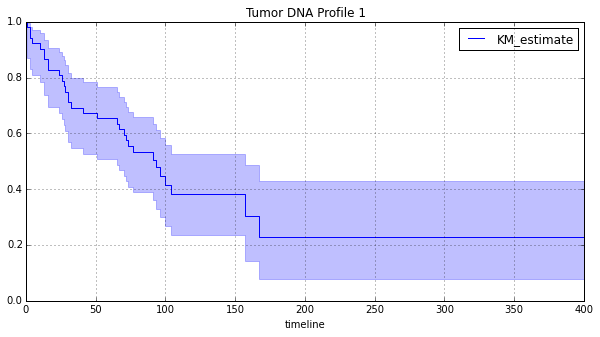Now we can convert this plot to an interactive Plotly object. However, we will have to augment the legend and filled area manually. Once we create a helper function, the process is simple.

Please see the Plotly Python user guide for more insight on how to update plot parameters.

Don't forget you can also easily edit the chart properties using the Plotly GUI interface by clicking the "Play with this data!" link below the chart.

In :
p = kmf.plot(ci_force_lines=True, title='Tumor DNA Profile 1 (95% CI)')

# Collect the plot object
kmf1 = plt.gcf()

def pyplot(fig, ci=True, legend=True):
# Convert mpl fig obj to plotly fig obj, resize to plotly's default
py_fig = tls.mpl_to_plotly(fig, resize=True)

# Add fill property to lower limit line
if ci == True:
style1 = dict(fill='tonexty')
# apply style
py_fig['data'].update(style1)

# Change color scheme to black
py_fig['data'].update(dict(line=Line(color='black')))

# change the default line type to 'step'
py_fig['data'].update(dict(line=Line(shape='hv')))
# Delete misplaced legend annotations
py_fig['layout'].pop('annotations', None)

if legend == True:
# Add legend, place it at the top right corner of the plot
py_fig['layout'].update(
showlegend=True,
legend=Legend(
x=1.05,
y=1
)
)

# Send updated figure object to Plotly, show result in notebook
return py.iplot(py_fig)

pyplot(kmf1, legend=False)

Out:

# Multiple Types¶

### Using R¶

Many times there are different groups contained in a single dataset. These may represent categories such as treatment groups, different species, or different manufacturing techniques. The type variable in the tongues dataset describes a patients DNA profile. Below we define a Kaplan-Meier estimate for each of these groups in R and Python.

In :
%%R

surv.fit2 <- survfit( Surv(time, delta) ~ type)

p <- ggsurv(surv.fit2) +
ggtitle('Lifespans of different tumor DNA profile') + theme_bw()
p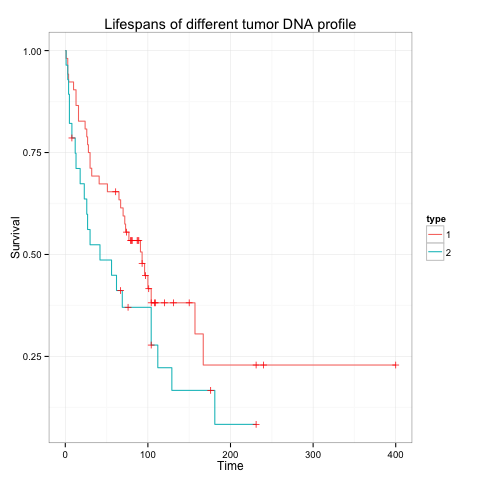Convert to a Plotly object.

In :
#%R ggplotly(plt)

%R p <- plot.ly("https://plotly.com/~rmdk/173/lifespans-of-different-tumor-dna-profile/")
# pass object to python kernel
%R -o p

# Render HTML
HTML(p)

Out:

### Using Python¶

In :
f2 = tongue.type==2
T2 = tongue[f2]['time']
C2 = tongue[f2]['delta']

ax = plt.subplot(111)

kmf.fit(T, event_observed=C, label=['Type 1 DNA'])
kmf.survival_function_.plot(ax=ax)
kmf.fit(T2, event_observed=C2, label=['Type 2 DNA'])
kmf.survival_function_.plot(ax=ax)

plt.title('Lifespans of different tumor DNA profile')

kmf2 = plt.gcf()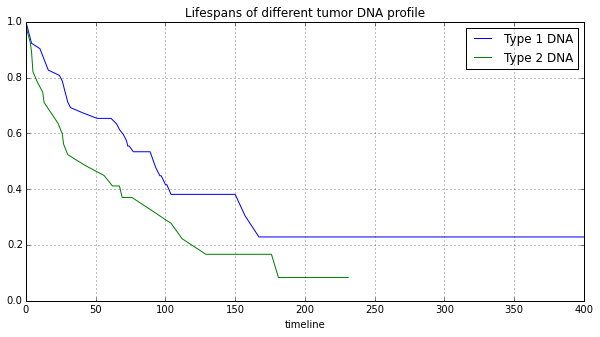Convert to a Plotly object.

In :
pyplot(kmf2, ci=False)

Out:

# Testing for Difference¶

It looks like DNA Type 2 is potentially more deadly, or more difficult to treat compared to Type 1. However, the difference between these survival curves still does not seem dramatic. It will be useful to perform a statistical test on the different DNA profiles to see if their survival rates are significantly different.

Python's lifelines contains methods in lifelines.statistics, and the R package survival uses a function survdiff(). Both functions return a p-value from a chi-squared distribution.

It turns out these two DNA types do not have significantly different survival rates.

### Using R¶

In :
%%R
survdiff(Surv(time, delta) ~ type)

Call:
survdiff(formula = Surv(time, delta) ~ type)

N Observed Expected (O-E)^2/E (O-E)^2/V
type=1 52       31     36.6     0.843      2.79
type=2 28       22     16.4     1.873      2.79

Chisq= 2.8  on 1 degrees of freedom, p= 0.0949


### Using Python¶

In :
from lifelines.statistics import logrank_test
summary_= logrank_test(T, T2, C, C2, alpha=99)

print summary_

<lifelines.StatisticalResult:
Results
df: 1
alpha: 99
t 0: -1
test: logrank
null distribution: chi squared

__ p-value ___|__ test statistic __|____ test result ____|__ is significant __
0.09487 |              2.790 |  Cannot Reject Null |       False
>


# Estimating Hazard Rates¶

### Using R¶

To estimate the hazard function, we compute the cumulative hazard function using the Nelson-Aalen estimator, defined as:

$$\hat{\Lambda} (t) = \sum_{t_i \leq t} \frac{d_i}{n_i}$$

where $d_i$ is the number of deaths at time $t_i$ and $n_i$ is the number of susceptible individuals. Both R and Python modules use the same estimator. However, in R we will use the -log of the Fleming and Harrington estimator, which is equivalent to the Nelson-Aalen.

In :
%%R

haz <- Surv(time[type==1], delta[type==1])
haz.fit  <- summary(survfit(haz ~ 1), type='fh')

x <- c(haz.fit$time, 250) y <- c(-log(haz.fit$surv), 1.474)
cum.haz <- data.frame(time=x, cumulative.hazard=y)

p <- ggplot(cum.haz, aes(time, cumulative.hazard)) + geom_step() + theme_bw() +
ggtitle('Nelson-Aalen Estimate')
p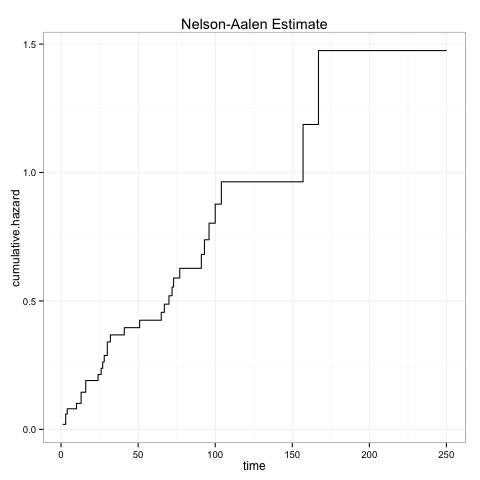In :
%R p <- plot.ly("https://plotly.com/~rmdk/185/cumulativehazard-vs-time/")
# pass object to python kernel
%R -o p

# Render HTML
HTML(p)

Out:

### Using Python¶

In :
from lifelines.estimation import NelsonAalenFitter

naf = NelsonAalenFitter()
naf.fit(T, event_observed=C)

naf.plot(title='Nelson-Aalen Estimate')

Out:
<matplotlib.axes._subplots.AxesSubplot at 0x1131833d0>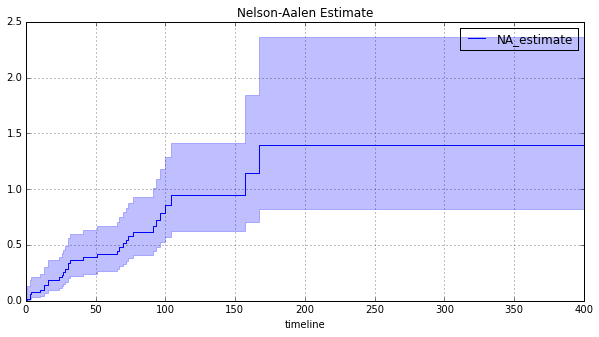In :
naf.plot(ci_force_lines=True, title='Nelson-Aalen Estimate')
py_p = plt.gcf()

pyplot(py_p, legend=False)

Out: